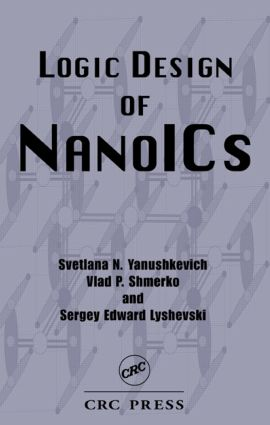Logic Design of NanoICS

1st Edition

CRC Press

488 pages | 251 B/W Illus.

Purchasing Options:\$ = USD
Hardback: 9780849327667
pub: 2004-10-28
SAVE ~\$35.00
\$175.00
\$140.00
x
eBook (VitalSource) : 9781315220437
pub: 2017-12-19
from \$28.98

FREE Standard Shipping!

Description

Today's engineers will confront the challenge of a new computing paradigm, relying on micro- and nanoscale devices. Logic Design of NanoICs builds a foundation for logic in nanodimensions and guides you in the design and analysis of nanoICs using CAD. The authors present data structures developed toward applications rather than a purely theoretical treatment.

Requiring only basic logic and circuits background, Logic Design of NanoICs draws connections between traditional approaches to design and modern design in nanodimensions. The book begins with an introduction to the directions and basic methodology of logic design at the nanoscale, then proceeds to nanotechnologies and CAD, graphical representation of switching functions and networks, word-level and linear word-level data structures, 3-D topologies based on hypercubes, multilevel circuit design, and fault-tolerant computation in hypercube-like structures. The authors propose design solutions and techniques, going beyond the underlying technology to provide more applied knowledge.

This design-oriented reference is written for engineers interested in developing the next generation of integrated circuitry, illustrating the discussion with approximately 250 figures and tables, 100 equations, 250 practical examples, and 100 problems. Each chapter concludes with a summary, references, and a suggested reading section.

Reviews

". . . interesting and full of information reported in a unified form . . ."

– Gaetano Palumbo, in IEEE Circuits & Devices Magazine, Jan/ Feb 2006, Vol. 22, No. 1

PREFACE

ACKNOWLEDGEMENTS

INTRODUCTION

Progress From Micro- to Nanoelectronics

Towards Computer-Aided Design of NanoICs

Methodology

Example: Hypercube Structure of Hierarchical FPGA

Summary

Problems

References

NANOTECHNOLOGIES

Nanotechnologies

Nanoelectronic Devices

Digital Nanoscale Circuits: Gates vs. Arrays

Molecular Electronics

Scaling and Fabrication

Summary

Problems

References

Graphs

Data Structures for Switching Functions

Sum-of-Products Expressions

Shannon Decision Trees and Diagrams

Reed-Muller Expressions

Decision Trees and Diagrams

Arithmetic Expressions

Decision Trees and Diagrams

Summary

Problems

References

WORD-LEVEL DATA STRUCTURES

Word-level Data Structures

Word-level Arithmetic Expressions

Word-level Sum-of-Products Expressions

Word-level Reed-Muller Expressions

Summary

Problems

References

NANOSPACE AND HYPERCUBE-LIKE DATA STRUCTURES

Spatial Structures

Hypercube Data Structure

Assembling of Hypercubes

N-Hypercube Definition

Degree of Freedom and Rotation

Coordinate Description

N-Hypercube Design for n > 3 Dimensions

Embedding a Binary Decision Tree in N-Hypercube

Assembling

Spatial Topological Measurements

Summary

Problems

References

NANODIMENSIONAL MULTILEVEL CIRCUITS

Graph-Based Models in Logic Design of Multilevel Networks

Library of N-Hypercubes for Elementary Logic Functions

Hybrid Design Paradigm: N-Hypercube and DAG

Manipulation of N-Hypercubes

Numerical Evaluation of 3-D Structures

Summary

References

LINEAR WORD-LEVEL MODELS OF MULTILEVEL CIRCUITS

Linear Expressions

Linear Arithmetic Expressions

Linear Arithmetic Expressions of Elementary Functions

Linear Decision Diagrams

Representation of a Circuit Level by Linear Expression

Linear Decision Diagrams for Circuit Representation

Technique for Manipulating the Coefficients

Linear Word-level Sum-of-Products Expressions

Linear Word-level Reed-Muller Expressions

Summary

Problems

References

EVENT-DRIVEN ANALYSIS OF HYPERCUBE-LIKE TOPOLOGY

Formal Definition of Change in a Binary System

Computing Boolean Differences

Models of Logic Networks in Terms of Change

Matrix Models of Change

Models of Directed Changes in Algebraic Form

Local Computation Via Partial Boolean Difference

Generating Reed-Muller Expressions by Logic Taylor Series

Arithmetic Analogs of Boolean Differences and Logic Taylor Expansion

Summary

Problems

References

NANODIMENSIONAL MULTIVALUED CIRCUITS

Introduction to Multivalued Logic

Spectral Technique

Multivalued Decision Trees and Decision Diagrams

Concept of Change in Multivalued Circuits

Generation of Reed-Muller Expressions

Linear Word-level Expressions of Multivalued Functions

Linear Nonarithmetic Word-level Representation of Multivalued Functions

Summary

Problems

References

PARALLEL COMPUTATION IN NANOSPACE

Data Structures and Massive Parallel Computing

Arrays

Linear Systolic Arrays for Computing Logic Functions

Computing Reed-Muller Expressions

Computing Boolean Differences

Computing Arithmetic Expressions

Computing Walsh Expressions

Tree-Based Network for Manipulating a Switching Function

Hypercube Arrays

Summary

Problems

References

FAULT-TOLERANT COMPUTATION

Definitions

Probabilistic Behavior of Nanodevices

Neural Networks

Stochastic Computing

Von Neumann's Model on Reliable Computation with Unreliable Components

Faulty Hypercube-Like Computing Structures

Summary

References

INFORMATION MEASURES IN NANODIMENSIONS

Information-Theoretical Measures at Various Levels of Design in Nanodimensions

Information-Theoretical Measures in Logic Design

Information Measures of Elementary Switching Functions

Information-Theoretical Measures in Decision Trees

Information Measures in the N-Hypercube

Information-Theoretical Measures in Multivalued Functions

Summary

Problems

References

INDEX